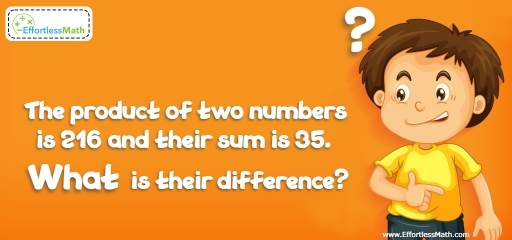# Ratio, Proportion and Percentages Puzzle – Challenge 30

This is a perfect math puzzle for those who love challenging their brain. The solution is also provided.## Challenge:

The product of two numbers is 216 and their sum is 35. What is their difference?

### The Absolute Best Book to challenge your Smart Student!

Let x be the first number and y the next number. Therefore:
$$xy = 216$$ and $$x + y = 35$$. Solve the second equation for x:
$$x + y = 35 → x = 35 – y$$
Replace the value of x in the second equation with x in the first equation.
$$xy = 216 → (35 – y) y = 216 →35y – y^2 = 216 →$$
$$y^2 – 35y + 216 = 0$$
Factorize the polynomial: $$(y – 8) (y – 27) = 0$$
$$y = 8$$ or $$y = 27$$
The difference is 19.

The Absolute Best Books to Ace Algebra

### What people say about "Ratio, Proportion and Percentages Puzzle – Challenge 30 - Effortless Math: We Help Students Learn to LOVE Mathematics"?

No one replied yet.

X
45% OFF

Limited time only!

Save Over 45%

SAVE $40 It was$89.99 now it is \$49.99